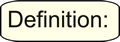Quadratics and Identities MathBitsNotebook.com Terms of Use   Contact Person: Donna RobertsAn equation that is true for every value of the variable is called an identity.

To show that an equation is an identity:
Start with either side of the equation and show that it can algebraically be changed into the other side. Or start with both sides of the equation and show that they both can be changed into the same algebraic expression.You may be asked to verify (show, prove) that a known "rule" is an identity (always true). You may, or may not, be asked to supply "justification" for your work.

1. Show, with justification, that (a - b)(a + b) = a2 - b2 is an identity.

 (a - b)(a + b) = a2 + ab - ab - b2 by the distributive property. = a2 - b2 by combining like terms. (a - b)(a + b) = a2 - b2 Identity! (always true)You may be asked to verify (show, prove) that any algebraic equation is an identity.

NOTE:
If you are asked to show that an equation is an identity, you may assume that the equation IS an identity. If you are asked to determine whether an equation is an identity, be careful as the answer may be that it is not an identity.

 2. Show that x2 + 8(x + 2) = (x + 4)2 is an identity. x2 + 8(x + 2) = x2 + 8x + 16 (distribute)                      = (x + 4)(x + 4) (factor)                      = (x + 4)2 (multiply) x2 + 8(x + 2) = (x + 4)2 (Is an identity.) 3. Determine whether b(2b + a + 1) + a = (a + b)(2b + 1) is an identity. b(2b + a + 1) + a = (a + b)(2b + 1) 2b2 + ab + b + a = 2ab + a + 2b2 + b 2b2 + ab + b + a = 2b2 + 2ab + b + a ab = 2ab 1 = 2 (FALSE) This is not an identity.

4. Determine whether x2 + y2 = ( x + yi )( x - yi ) is an identity.
("i " is the imaginary unit.)
 ( x + yi )( x - yi ) = x2 - xyi + xyi - (yi)2 multiplication (distribution) = x2 - (yi)2 combine like terms = x2 - y2• i2 property of exponents = x2 - y2• (-1) i2 = -1 = x2 + y2 multiplication by (-1) x2 + y2 = ( x + yi )( x - yi ) is an identity.You may be asked to "check" someone's work to see if they properly showed the existence of an identity.

Keith gave the following justification that (3x - 2)(x - 3)2 = 3x3 - 20x2 + 15x - 18 is an identity.
Is this correct? If not, where is the error?
 5. (3x - 2)(x - 3)2 Left side of equation. Step 1: = (3x - 2)(x - 3)(x - 3) Interpretation of squaring. Step2: = (3x - 2)(x2 - 3x - 3x + 9) Multiplication (distribution). Step 3: = (3x - 2)(x2 - 6x + 9) Combining like terms. Step 4: = 3x3 - 18x2 + 27x - 2x2 - 12x - 18 Multiplication (distribution). Step 5: = 3x3 - 20x2 + 15x - 18 Combining like terms. (3x - 2)(x - 3)2 = 3x3 - 20x2 + 15x - 18     Makes it an identity.

Keith made an error in Step 4. His fifth term should be +12x (not -12x).
(3x - 2)(x - 3)2 does not equal 3x3 - 20x2 + 15x - 18 for all values of x and is NOT an identity.
[Yes, Keith's equation is TRUE when x = 0, but zero alone is insufficient to show the equation to be an "identity".]